## Transform equation to reach a solved pattern

### Quick description

Apply reversible transformations to the equation which give logically equivalent equations, until one side (or an important chunk) looks like a known, "solved" pattern.

### General discussion

You should have reversible transformations in your formal algebra – transformations which create logically equivalent statements. In basic algebra, adding the same formula to both sides is an example; so is multiplying by a non-zero value.

You can also use some transformations which only have one-way logical implication, if you're careful. More.... (For instance, squaring both sides creates an equation with more solutions than the original equation; taking the square root of both sides creates one with fewer solutions. As long as you only add spurious solutions during your transformations, you may get a superset of the true set of solutions which you can cut down by other means. Alternatively, as long as you only remove solutions, you may get a useful result if you are trying for a single solution rather than all solutions.)

The core trick is to apply these transformations with the aim of arriving at a pattern which is already "solved", or at least "better".

This will usually involve manipulations which introduce extra terms and complication to the equation. Unless you know what pattern the writer is aiming for, these manipulations can appear utterly unmotivated in written proofs.

Try to capture all the instances of the variable you're trying to solve for or the part of the equation which is giving you trouble in the "solved pattern" side of the equation; if a few escape, the technique may still simplify your problem, but if lots escape, you won't be getting anywhere.

### Example 1: Completing the square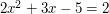The pattern we're looking for is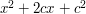, because this equals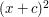, and we "know" how to solve the equation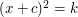by taking a square root.

So, to make our equation look more like that, divide both sides by 2: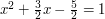We want to keep all the instances ofin the pattern side of the equation, so there's not much more we can do with the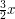term. So to match the pattern we try to make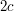equal. This leads to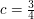and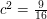. So add constants to both sides to make it look like the pattern: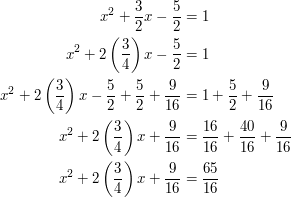We now match the pattern. We replace it with the equivalent "better" version: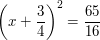We take the square root and arrive at the solutions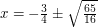.

### Example 2: The quadratic formula

Repeat the "completing the square" procedure for the generic quadratic equation, assuming non-zero.More... (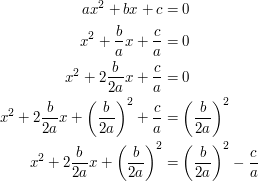Here, we've matched the pattern. Now apply it and tidy up: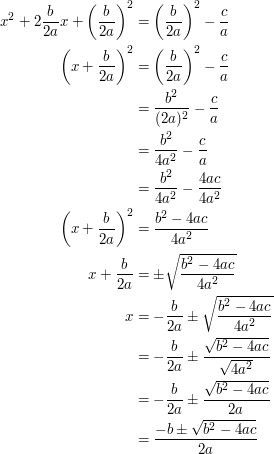)

### General discussion

There's a whole article about completing the square: Complete the square.

### Prerequisites

Some equations in your field should have been solved.

This trick applies most readily when the equation you are solving is essentially finding an inverse, where one direction is "easy" (forming a polynomial, differentiating) and you're trying to go the "hard" way (solving a polynomial, integrating). In this case there will be a list of solved equations, generated by going the "easy" way, and you are trying to tweak your equation so it looks like one of them.

### Example 3: Integration by parts

In general, to use integration by parts, we try to make the integral look like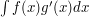, with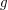known and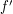simple – which is already an example of trying to rearrange to match a pattern.

But the derivation of the integration by parts formulas which make that pattern "good" is also a use of this same technique. The pattern we are looking for is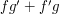, because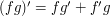. More... ( Let the unknown value of the integral be r(x). Then manipulate: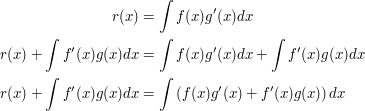We matched the "solved" pattern, now clean up using the Fundamental Theorem of Calculus.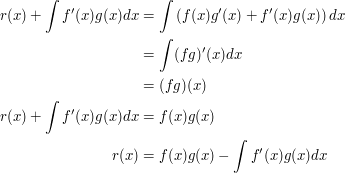)

When completing the square, all the "leftovers" outside the pattern were numbers which could be reduced by arithmetic. Here, unlike when completing the square, the transformations we made left leftovers outside the pattern which don't necessarily simplify. The technique of transforming to match a pattern is only useful when the leftovers are simpler in some sense than the starting equation, and integration by parts provides a good example of that.

### Example 4: Row reduction

When looking at a system of linear equations in several variables – or equivalently, an equation in matrices and vectors of the form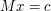– there's one pattern which is clearly easy to solve, namely the identity matrix: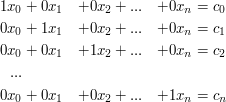There are also several reversible transformations available in this linear algebra, which do not change the solutions of the system: multiplying an equation (row) by a nonzero constant; adding a constant multiple of a row to another row; swapping two rows.

Row reduction is, simply, an algorithm which uses these transformations to make the system of equations look more and more like the desirable pattern. In fact it proceeds by "improving" one matrix entry at a time using the transformations. More... ( First row 1 is multiplied by a constant so that row 1 column 1 will become 1. Then an appropriate multiple of row 1 is added to each row below it so that the rest of column 1 will be 0. Next row 2 is multiplied by a constant so that row 2 column 2 will become 1. Then an appropriate multiple of row 2 is added to each row below it, so that column 2 is zeros from row 3 down. This is repeated until row n column n. Some descriptions stop there, with a "diagonal matrix" with zeros below and to the left of the main diagonal. But the full algorithm then clears the portion of column n above row n (by adding appropriate copies of row n, which is now in the form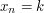), then the portion of column n-1 above row n-1, and so on until the identity matrix is reached. If there's a zero in a spot which should have a 1, you add another row which doesn't have a zero in that column to it before continuing.)

The algorithm doesn't always get you to the identity matrix – but the attempt is still helpful! If, during the process, there's a full column of zero coefficients, you have an irrelevant variable. If there's a full row of zeros equalling a zero constant, you had a redundant equation originally. If there's a full row of zeros equalling a nonzero constant, you have a contradictory system.

### General discussion

Row reduction is an example where trying to make an equation match the desired pattern can lead to interesting results even if you can't actually make it match that pattern. This is another positive effect of this trick; sometimes trying to arrange an equation to match a pattern will cause the "weird" or interesting part of the equation to pop out at you, when you see why it can't be made to match the pattern.

The following comments were made inline in the article. You can click on 'view commented text' to see precisely where they were made.

### Should "difficult" be changed

Should "difficult" be changed to "easy"?

### Corrected now

Blech, yes, I got stuff reversed. Fixing.

This describes one of the most fundamental and general mathematical techniques there is, one so basic that probably most math majors find it second nature already. As such, the description of it as a general technique may be most useful for lower-level pedagogy; failing to grasp this general technique can make math very hard for primary and secondary school students. However, I would love to have examples of its use at all levels and fields, since it is so general-purpose.

### Notion of bypass or T U T^-1

This is indeed one instance of one of the most general method useful in mathematics and all the sciences. Z. A. Melzak has devoted a whole book to this idea : "Bypasses" (Wiley, 1983) showing how it encompasses geometry, algebra, analysis, physics, chemistry, information theory, technology in general.

In this tricki entry, contributors have shown classical instances of how to find and build T (a transformation toward another form) motivated by the fact we know how to do U (the transformation/projection of the target form into needed information, here its solutions), mainly with algebraic objects.

One of the most important aspect of this method or trick is that it can be nested at will.

## Post new comment

(Note: commenting is not possible on this snapshot.)

Before posting from this form, please consider whether it would be more appropriate to make an inline comment using the Turn commenting on link near the bottom of the window. (Simply click the link, move the cursor over the article, and click on the piece of text on which you want to comment.)